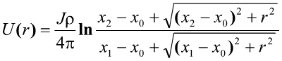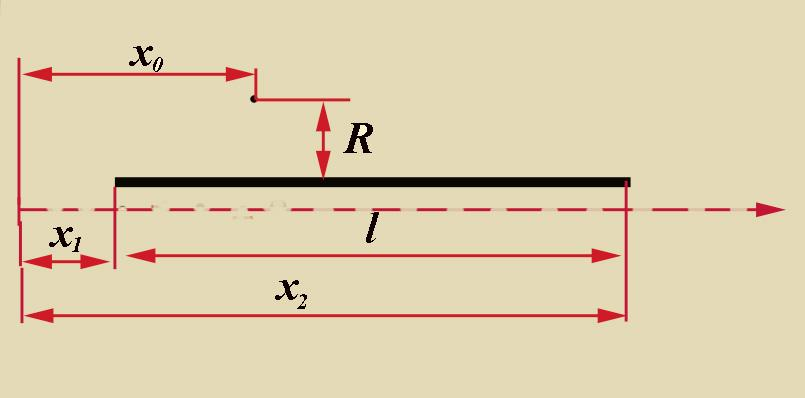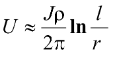# 3. Calculation technique

From the series of articles "Grounding resistance calculation is almost simple".

I don’t know why, but many people are confused by the question of how many times the conductivity of the best soil is less than the conductivity of the metal from which the grounding electrodes are made. The difference here is huge - in about a billion times. In such conditions there is no sense in considering the voltage drop on the grounding buses from the current spreading through them, and therefore, a potential at any point on the grounding device buses with a constant or slowly changing current can be considered the same. This greatly simplifies the calculation of potential. Focusing on grounding buses, the length of which is much greater than their radius, you can do with one single calculation formula. Assuming that the leakage current per unit length of the conductor J (linear leakage current) is constant over its entire length, the point potential in a homogeneous unlimited medium can be written as(6)To the formula for calculating the potential in the soil

As can be seen from the explanatory figure, x1 and x2 are the coordinates of the beginning and end of the bus (conductor) with current in the ground, on the coordinate axis parallel to this bus, x0 is the coordinate on the same axis of the point for which the potential is calculated, and r is the shortest distance from calculated point to the axis. The origin of the coordinate axis can be set arbitrarily, but it is more convenient to combine it with the edge of the bus (x1=0). The calculation point is most often located in the middle of one of the buses of the grounding device, best of all somewhere in its center. The calculation error associated with the arbitrariness of the choice of the calculation point is very little significant. If the calculated point is located on the surface of one of the buses, when calculating the potential from its current, the distance r is equal to the radius of the bus r0.

Another modification of formula (6) is convenient, which is obtained for x1=0, x2=l and 0= l/2, when the condition r << l is satisfied:(7)

It will certainly simplify the calculation of the potential component of the self-current of the grounding bus when r=r0

As you can see, there is not a word about load factors in the text.

Related Articles: